2016/08/05 22:55

# 翻译:非常详细易懂的法线贴图(Normal Mapping)

## 概述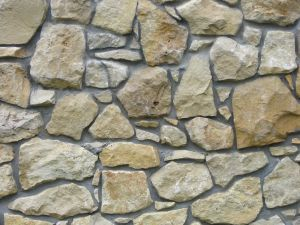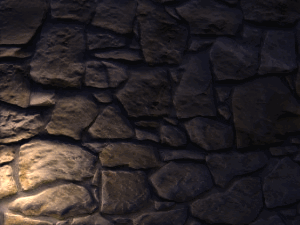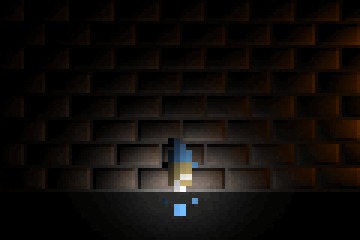## 介绍向量和法线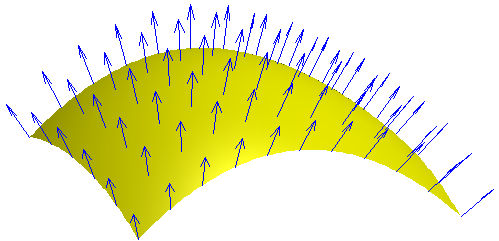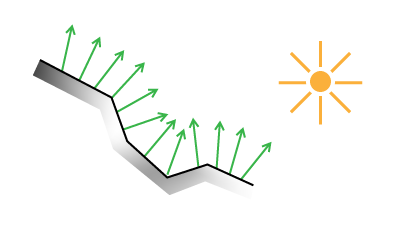法线贴图(Normal Mapping)是一个游戏编程技巧, 它允许我们渲染相同数目的多边形(例如低解析度的网格模型), 但是在计算光照时使用高解析度网格模型的法线. 这为我们带来更好的感受, 关于深度, 真实性和光滑度.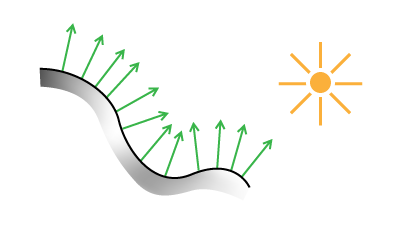(图像来自于这个出色的博客文章Making Worlds 3 - That's no Moon...)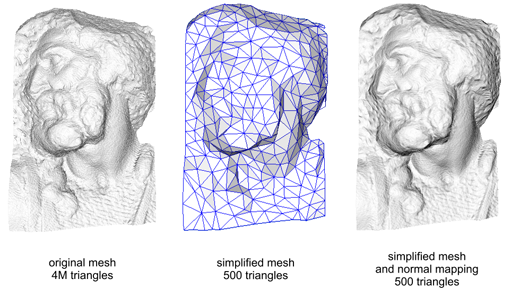## 对法线编码和解码

Color.rgb = Normal.xyz / 2.0 + 0.5;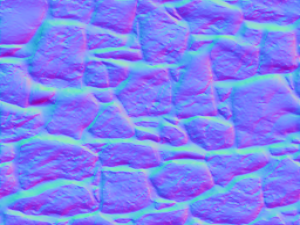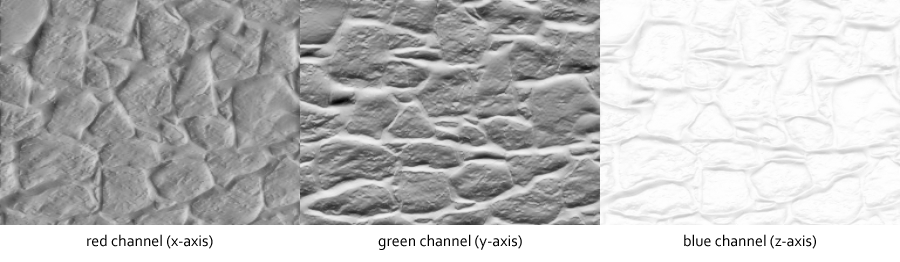//sample the normal map
NormalMap = texture2D(NormalMapTex, TexCoord);

//convert to range -1.0 to 1.0
Normal.xyz = NormalMap.rgb * 2.0 - 1.0;


## Lambertian 光照模型

N = normalize(Normal.xyz)
L = normalize(LightDir.xyz)

Diffuse = LightColor * max(dot(N, L), 0.0)

Ambient = AmbientColor * AmbientIntensity

Attenuation = 1.0 / (ConstantAtt + (LinearAtt * Distance) + (QuadraticAtt * Distance * Distance))

Intensity = Ambient + Diffuse * Attenuation

FinalColor = DiffuseColor.rgb * Intensity.rgb


• Normal-法线: 从法线图中解码得到的法线向量 XYZ.
• LightDir-光线方向: 从物体表面到光源位置的向量, 我们将会简单解释.
• Diffuse Color-漫射颜色: 纹理贴图的 RGB 颜色, 没有光.
• Diffuse-漫射: 跟Lambertian反射相乘的光线颜色, 这是我们光照等式的主要部分.
• Ambient-环境光: 处于阴影中的颜色和强度, 例如, 一个户外场景会有一个更亮的环境光强度, 比起一个暗淡灯光下的户内场景.
• Attenuation-衰减: 这是光线的随距离而降低, 例如, 当我们远离点光源时强度/亮度的损失. 有多种方法来计算衰减--对于我们的目标而言, 我们将会使用常量-线性-二次方衰减. 这里用3个系数来计算衰减, 我们可以改变它们来影响光线衰减的视觉效果.
• Intensity-强度: 我们阴影算法的强度--离1.0越近意味着有光, 离0.0越近意味着没有光.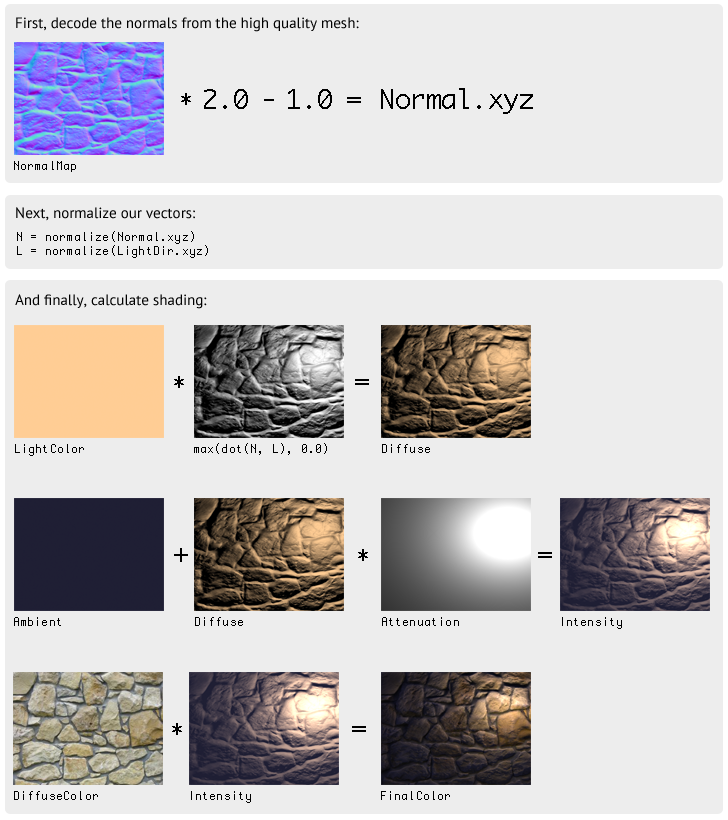## Java 例程public static final float DEFAULT_LIGHT_Z = 0.075f;
...
//Light RGB and intensity (alpha)
public static final Vector4f LIGHT_COLOR = new Vector4f(1f, 0.8f, 0.6f, 1f);

//Ambient RGB and intensity (alpha)
public static final Vector4f AMBIENT_COLOR = new Vector4f(0.6f, 0.6f, 1f, 0.2f);

//Attenuation coefficients for light falloff
public static final Vector3f FALLOFF = new Vector3f(.4f, 3f, 20f);


...

//update light position, normalized to screen resolution
float x = Mouse.getX() / (float)Display.getWidth();
float y = Mouse.getY() / (float)Display.getHeight();
LIGHT_POS.x = x;
LIGHT_POS.y = y;

//send a Vector4f to GLSL

//bind normal map to texture unit 1
glActiveTexture(GL_TEXTURE1);
rockNormals.bind();

//bind diffuse color to texture unit 0
glActiveTexture(GL_TEXTURE0);
rock.bind();

//draw the texture unit 0 with our shader effect applied
batch.draw(rock, 50, 50);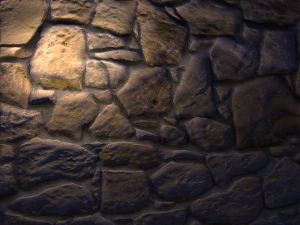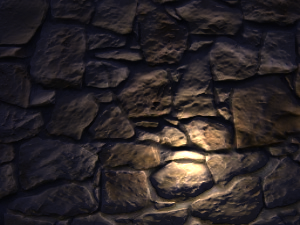### 片段着色器

//attributes from vertex shader
varying vec4 vColor;
varying vec2 vTexCoord;

//our texture samplers
uniform sampler2D u_texture;   //diffuse map
uniform sampler2D u_normals;   //normal map

uniform vec2 Resolution;      //resolution of screen
uniform vec3 LightPos;        //light position, normalized
uniform vec4 LightColor;      //light RGBA -- alpha is intensity
uniform vec4 AmbientColor;    //ambient RGBA -- alpha is intensity
uniform vec3 Falloff;         //attenuation coefficients

void main() {
//RGBA of our diffuse color
vec4 DiffuseColor = texture2D(u_texture, vTexCoord);

//RGB of our normal map
vec3 NormalMap = texture2D(u_normals, vTexCoord).rgb;

//The delta position of light
vec3 LightDir = vec3(LightPos.xy - (gl_FragCoord.xy / Resolution.xy), LightPos.z);

//Correct for aspect ratio
LightDir.x *= Resolution.x / Resolution.y;

//Determine distance (used for attenuation) BEFORE we normalize our LightDir
float D = length(LightDir);

//normalize our vectors
vec3 N = normalize(NormalMap * 2.0 - 1.0);
vec3 L = normalize(LightDir);

//Pre-multiply light color with intensity
//Then perform "N dot L" to determine our diffuse term
vec3 Diffuse = (LightColor.rgb * LightColor.a) * max(dot(N, L), 0.0);

//pre-multiply ambient color with intensity
vec3 Ambient = AmbientColor.rgb * AmbientColor.a;

//calculate attenuation
float Attenuation = 1.0 / ( Falloff.x + (Falloff.y*D) + (Falloff.z*D*D) );

//the calculation which brings it all together
vec3 Intensity = Ambient + Diffuse * Attenuation;
vec3 FinalColor = DiffuseColor.rgb * Intensity;
gl_FragColor = vColor * vec4(FinalColor, DiffuseColor.a);
}


### GLSL 分解

//RGBA of our diffuse color
vec4 DiffuseColor = texture2D(u_texture, vTexCoord);

//RGB of our normal map
vec3 NormalMap = texture2D(u_normals, vTexCoord).rgb;


//Delta pos
vec3 LightDir = vec3(LightPos.xy - (gl_FragCoord.xy / Resolution.xy), LightPos.z);

//Correct for aspect ratio
LightDir.x *= Resolution.x / Resolution.y;

//determine magnitude
float D = length(LightDir);


vec3 N = normalize(NormalMap * 2.0 - 1.0);
vec3 L = normalize(LightDir);


//Pre-multiply light color with intensity
//Then perform "N dot L" to determine our diffuse term
vec3 Diffuse = (LightColor.rgb * LightColor.a) * max(dot(N, L), 0.0);


vec3 Ambient = AmbientColor.rgb * AmbientColor.a;


float Attenuation = 1.0 / ( Falloff.x + (Falloff.y*D) + (Falloff.z*D*D) );


vec3 Intensity = Ambient + Diffuse * Attenuation;
vec3 FinalColor = DiffuseColor.rgb * Intensity;
gl_FragColor = vColor * vec4(FinalColor, DiffuseColor.a);


## 抓住你了(Gotchas)

• 在我们的实现中, LightDirattenuation 依赖于分辨率. 这意味着更改分辨率会影响我们的光的衰减. 根据你的游戏，不同的实现上分辨率无关可能是必需的.
• 一个必须处理的常见问题, 关于你游戏的 Y 坐标系和你所采用的法线图生成程序(例如 CrazyBump)之间的差异. 一些程序允许你导出一个翻转了Y轴的法线图. 下面的图片展示了这个问题: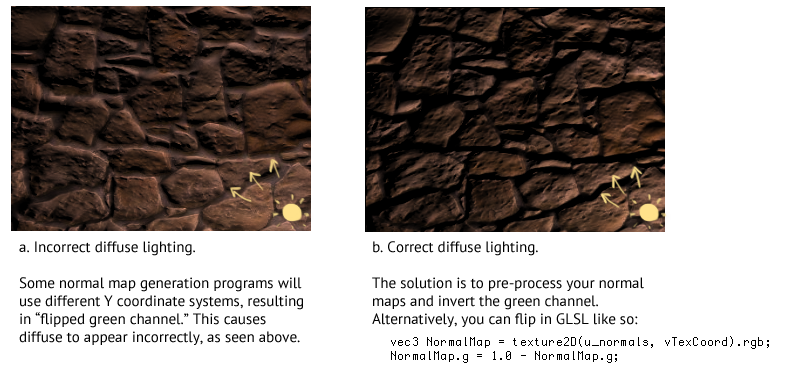## 多光源

vec3 Sum = vec3(0.0);
for (... each light ...) {
... calculate light using our illumination model ...
Sum += FinalColor;
}
gl_FragColor = vec4(Sum, DiffuseColor.a);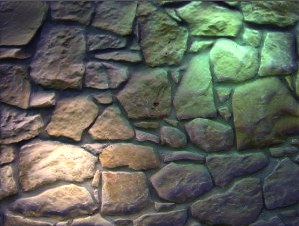## 生成法线图

### Blender工具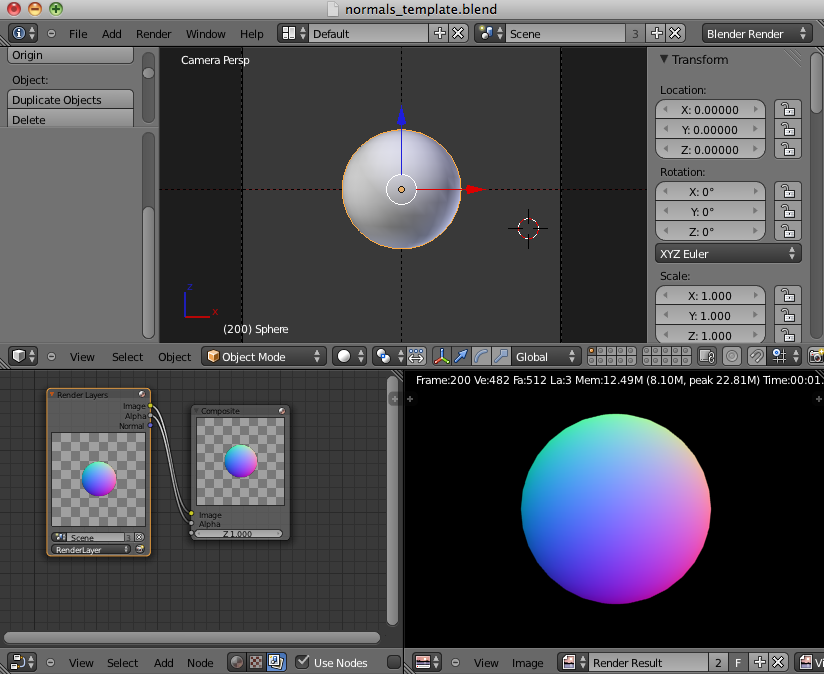## 其他 APIs0 评论
1 收藏
1